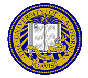# Notes for November 1, 1999

1. Greetings and Felicitations!
2. Puzzle of the Day
3. Public-Key Cryptography
1. Basic idea: 2 keys, one private, one public
2. Cryptosystem must satisfy:
1. given public key, CI to get private key;
2. cipher withstands chosen plaintext attack;
3. encryption, decryption computationally feasible [note: commutativity not required]
4. Benefits: can give confidentiality or authentication or both
4. Use of PKC
1. Normally used as key interchange system to exchange secret keys (cheap)
2. Then use secret key system (too expensive to use PKC for this)
5. RSA
1. Provides both authenticity and confidentiality
2. Go through algorithm:
Idea: C = Me mod n, M = Cd mod n, with ed mod PHI(n) = 1.
Proof: MPHI(n) mod n = 1 [by Fermat's theorem as generalized by Euler]; follows immediately from ed mod PHI(n) = 1.
Public key is (e, nn); private key is d. Choose n = pq; then PHI(n) = (p-1)(q-1).
3. Example:
p = 5, q = 7; n = 35, PHI(n) = (5-1)(7-1) = 24. Pick d = 11. Then de mod PHI(n) = 1, so choose e = 11. To encipher 2, C = Me mod n = 211 mod 35 = 2048 mod 35 = 18. M = Cd mod n = 1811 mod 35 = 18.
4. Example:
p = 53, q = 61, n = 3233, PHI(n) = (53-1)(61-1) = 3120. Take d = 791; then e = 71. Encipher M = RENAISSANCE: A = 00, B = 01, ..., Z = 25, blank = 26. Then:
M = RE NA IS SA NC Eblank = 1704 1300 0818 1800 1302 0426
C = (1704)71 mod 3233 = 3106; etc. = 3106 0100 0931 2691 1984 2927
6. Authentication:
1. validating client (user) identity
2. validating server (system) identity
3. validating both (mutual authentication)
7. Basis
1. What you know
2. What you have
3. What you are
1. How UNIX does selection
2. Problem: common passwords; Go through Morris and Thompson; Klein and mine, etc.
3. May be pass phrases: goal is to make search space as large as possible and distribution as uniform as possible
4. Other ways to force good password selection: random, pronounceable, computer-aided selection
5. Go through problems, approaches to each, esp. proactiveSend email to cs153@csif.cs.ucdavis.edu.

Department of Computer Science
University of California at Davis
Davis, CA 95616-8562